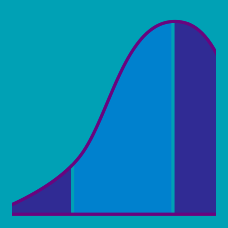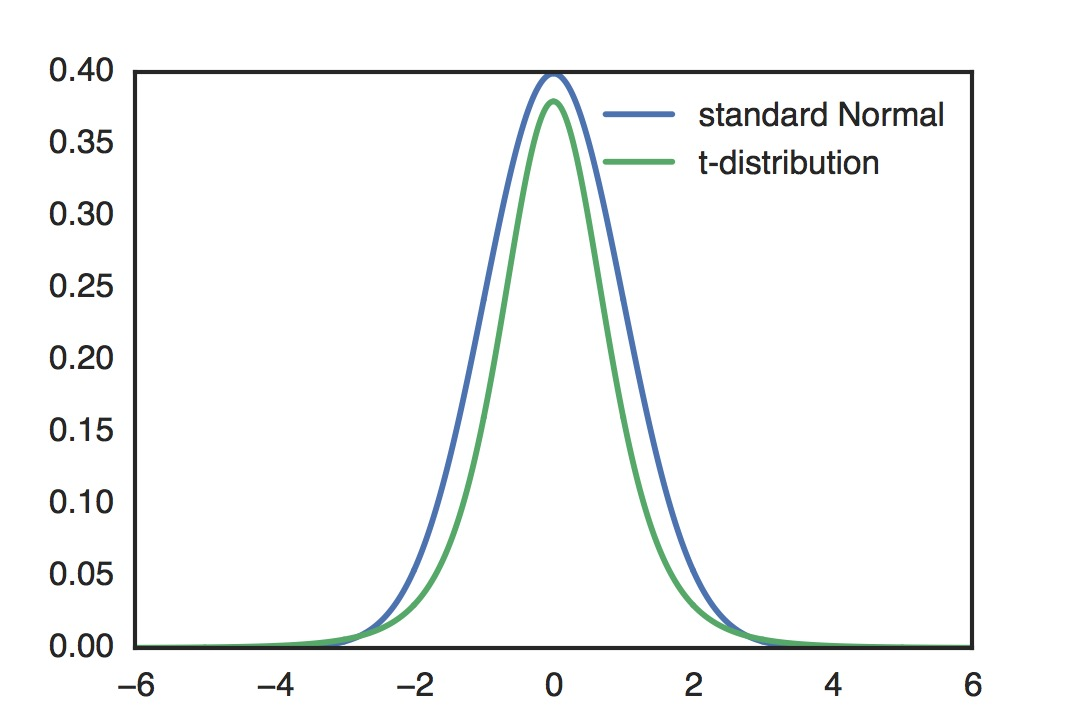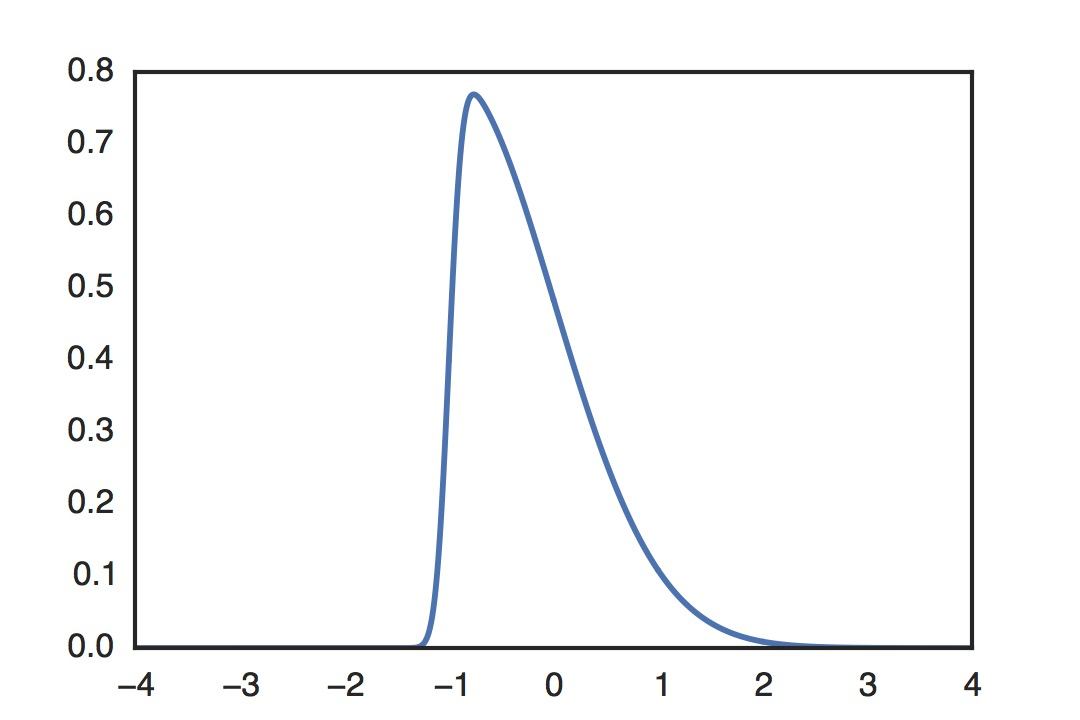Probability

# Higher Moments Warmup

True or false: If two distributions have the same mean and standard deviation, they must be the same.

Scores on a certain test have a mean of 75 and standard deviation of 5. Out of 100 random test papers, how many (rounded to the nearest integer) do we expect to have a score of at least 85?

Note: We cannot assume the distribution of scores is normal or approximately normal, we only know its mean and standard deviation.

At a certain company, the distribution of salaries is unimodal and skewed right. The company wants to entice outside workers to join, and thus wants to provide a measure of what a typical employee earns that is as high as possible. Which should they use?Both the distributions depicted in the graph have a mean of 0 and a standard deviation of 1. Which one has higher kurtosis?

Note: The kurtosis of some random variable $X$ with standard deviation 1 is $\mathbb{E}[(X-\mu_X)^4].$Consider the distribution with probability density function depicted in the graph. What is its skew?

Note: The skew of a random variable $X$ has the same sign as $\mathbb{E}[(X-\mu_X)^3].$

×